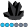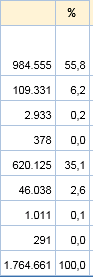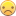Jaheuk
Obsidian | Level 7

## PROC TABULATE

dear all,

using <colpctsum> I get the percentage of a column with amounts.

great but HOW do I get tha value itself   ( amount * colpctsum )  ??

H.

1 ACCEPTED SOLUTION

Accepted Solutions

## Re: PROC TABULATE

Hi @Jaheuk

Totally agree with @Kurt_Bremser984.555 IS already 55,8% of 1.764.661.

But if you think that makes sense in your context, you can try this to get your report:

``````data have;
input amount;
datalines;
984555
109331
2933
378
620125
46038
1011
291
;
run;

proc sql;
create table want as
select a.amount,
a.amount / b.total as percent format=percent8.1,
a.amount * calculated percent as value
from have as a, (select sum(amount) as total from have) as b;
quit;

proc print data=want noobs;
sum amount percent value;
run;``````4 REPLIES 4

## Re: PROC TABULATE

Hi @Jaheuk

Could you please provide some sample data as well as the desired output?

Thank you!Jaheuk
Obsidian | Level 7

## Re: PROC TABULATE55,8 %  of amount 984.555 = 549.283 euro, so an extra column with this value

I googled a lot, but I think it is not possible to add a calculated column## Re: PROC TABULATE

984.555 IS already 55,8% of 1.764.661, so applying the percentage again makes no sense.

## Re: PROC TABULATE

Hi @Jaheuk

Totally agree with @Kurt_Bremser984.555 IS already 55,8% of 1.764.661.

But if you think that makes sense in your context, you can try this to get your report:

``````data have;
input amount;
datalines;
984555
109331
2933
378
620125
46038
1011
291
;
run;

proc sql;
create table want as
select a.amount,
a.amount / b.total as percent format=percent8.1,
a.amount * calculated percent as value
from have as a, (select sum(amount) as total from have) as b;
quit;

proc print data=want noobs;
sum amount percent value;
run;``````Discussion stats
• 4 replies
• 531 views
• 0 likes
• 3 in conversation